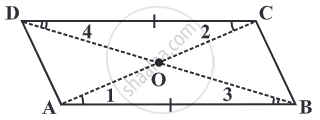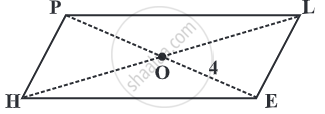The diagonals of a parallelogram bisect each other.

Given: ABCD is a parallelogram.To Prove: AO = OC and BO = OD.

Construction: Draw diagonal AC and diagonal BD.

Proof:

ABCD is a parallelogram.
∴ AB || DC and AD || BC

Now,
AB || DC, AC is the transversal intersecting them at A and C respectively.
∠BAC = ∠DCA                       ........(Alternate interior angles)
Thus, ∠BAO = ∠ DCO            .........(1)

As, AB || DC and BD is the transversal intersecting them at B and D respectively.
∠ABD = ∠CDB                    ........(Alternate interior angles)
So, ∠ABO = ∠CDO   ...(2)

In △ AOB and △ COD,
∠ DCO ≅ ∠BAO     .....(Pair of alternate interior angles)(1)
∠ CDO ≅ ∠ABO     .....(Pair of alternate interior angles)(2)

side DC = side AB ....(parallel side)

by ASA congruency condition,

△ AOB ≅ △ COD.

This gives, AO = CO and BO = DO.....(C.P.C.T)

Hence proved.

Example

In given Fig, HELP is a parallelogram. (Lengths are in cms). Given that OE = 4 and HL is 5 more than PE? Find OH.If OE = 4 then OP also is 4. .......(The diagonals of a parallelogram bisect each other.)

So, PE = 8,

Therefore, HL = 8 + 5 = 13

Hence, OH = 1/2 xx 13 = 6.5 cms.

If you would like to contribute notes or other learning material, please submit them using the button below.

Shaalaa.com

To Prove that the Diagonals of a Parallelogram Bisect Each Other [00:06:49]
S
0%